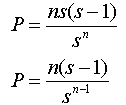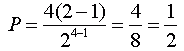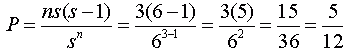From: Kalyan

I am a kind of person who do maths & statistics because I love these subjects. I came to know about your site from internet. Right now I am working on probability & number theory.

Q. When four people toss fair coins, what is the probability that in a given toss there will be one "odd man". That is one person whose coin does not have the same out comes as that of any other members?

Hi Kalyan.

We can easily write out all the possibilities and just identify how many there are with an "odd man" and divide that by the total number of possibilities:

 HHHH HTHH THHH TTHH HHHT HTHT THHT TTHT HHTH HTTH THTH TTTH HHTT HTTT THTT TTTT

So you can just read off the probability in this case, but what about a more general approach where the numbers are too big to use this simplistic approach?

Since each toss (and coin) is "fair", there is an equal likelihood of it turning out as heads or tails. This means there are two equal possibilities. Let s = 2.

Each toss is entirely independent of the others. That means the outcome on one toss has no bearing on the outcome of another toss. There are four independent tosses, so let n = 4.

Now the number of different four-toss outcomes is the number of choices for each toss multiplied together. This is sn .

That gives us the total (because 24 = 16, just as in our table). Now we can take a look at the events we really want to count: how many have an "odd man" out. We can interpret this as meaning that everyone except one person gets the same outcome.

Let's say that n-1 people get heads. How many choices does the last toss have, if it cannot be the same?

It could be anyone who gets it, so that means there are n possible people who didn't throw heads. How many non-heads outcomes can one toss have? s-1.

That means the number of four-toss outcomes with everyone getting heads except for one person is n(s-1). But this is only one of the s cases, so really there are s times this many odd-man out situations. That makes the total number of equally-likely outcomes ns(s-1). The probability, P, is this divided by the total sn:Let's check this with the original four-coins question:So the formula we came up with works for this situation. Let's test it on one other situation. Let's say 3 people roll fair six-sided dice (one each). What is the likelihood of an "odd man" situation?

In this case, n = 3 people and s = 6 sides, so we predict:Is this accurate? The numbers are still small enough for us to write out the possibilities:

 111 112 113 114 115 116 121 122 123 124 125 126 131 132 133 134 135 136 141 142 143 144 145 146 151 152 153 154 155 156 161 162 163 164 165 166

 211 212 213 214 215 216 221 222 223 224 225 226 231 232 233 234 235 236 241 242 243 244 245 246 251 252 253 254 255 256 261 262 263 264 265 266
 311 312 313 314 315 316 321 322 323 324 325 326 331 332 333 334 335 336 341 342 343 344 345 346 351 352 353 354 355 356 361 362 363 364 365 366

 411 412 413 414 415 416 421 422 423 424 425 426 431 432 433 434 435 436 441 442 443 444 445 446 451 452 453 454 455 456 461 462 463 464 465 466
 511 512 513 514 515 516 521 522 523 524 525 526 531 532 533 534 535 536 541 542 543 544 545 546 551 552 553 554 555 556 561 562 563 564 565 566

 611 612 613 614 615 616 621 622 623 624 625 626 631 632 633 634 635 636 641 642 643 644 645 646 651 652 653 654 655 656 661 662 663 664 665 666

If you add up the yellows, you'll find 15/36 of each table is yellow. This agrees with the formula we made.

Hope this helps!
Stephen La Rocque.